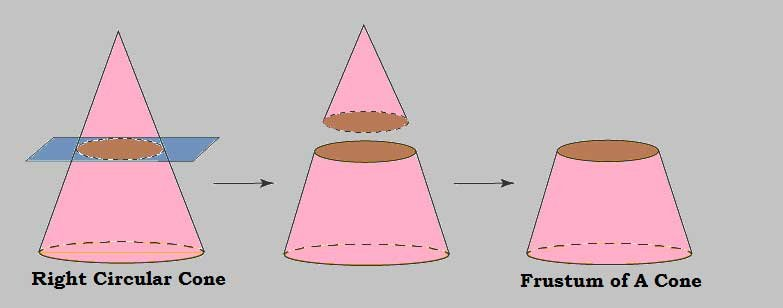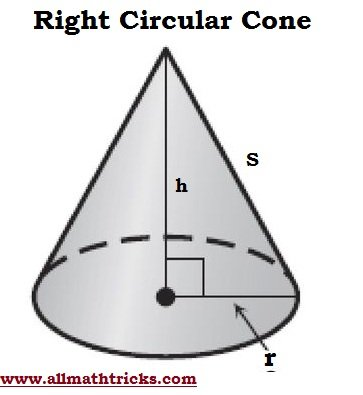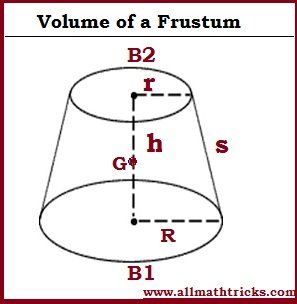# Frustum of cone formulas with examples | Surface area and Volume

## Conical Frustum Formulas – Slant height,Volume, Lateral, Base and Total surface areas

Frustum of A Cone

When cut a lower part of the right circular cone is called a frustum. We need to cut the right circular cone with a to cut it horizontally or parallel to the base of the cone, then the solid shape between the base and plane of the cone is called the frustum of a cone.Formulas of Right Circular ConeVolume of a Cone = VBase Area of a coneLateral surface area a coneTotal Surface area of a coneSlant height of a cone### Frustum of cone formulas – Volume of a Frustum of  Cone

The volume of a frustum can be defined as a the quantity of matter that it can hold in the space that is present inside it .Frustum of a cone of altitude “h” with a large base radius “R” and small base radius “r” . Slant heights “s” of the frustum and the cone.

Altitude ( h) is the perpendicular distance between the bases of a frustum of a cone.

Volume of a Frustum of a ConeFrustum volume can be calculated using its height and the areas of its bases. Let us consider a frustum large base areas “B1” and small base area “B2” and height “h” and . Then its volume is calculated by using the following formula

Volume of a Frustum of a ConeGenerator or Slant height of frustum of cone formula

The distance measured along the lateral face of the frustum is called slant height of the same

The Slant of the Frustum of a Cone (S)h= height of the Frustum cone

Slant height and Height of the cone which the frustum is a part of that cone.

Slant height of the cone of which the frustum is a part =Height of the cone of which the frustum is a part =### Frustum of cone formulas – Surface area of a Frustum of Cone

What Is the Surface Area of Frustum?

The surface area of a frustum area is calculated by the sum of areas of its faces and is measured in square units.

With respect to the frustum, there are having two types of surface areas.

1. Lateral Surface Area (LSA) or Curved Surface Area (CSA) for the frustum of a cone

2. Total Surface Area (TSA) for the frustum of a cone.

The curved surface area or lateral surface area of a frustum of a cone is the area of its curved face.

Frustum of a cone of height “h” with a large base radius “R” and small base radius “r” and “s” slant height of the frustum.Curved surface area of frustum of cone formula ( LSA or CSA)Slant height of the a frustum =The curved (or lateral) surface area of any frustum can be calculated using the circumferences  (or perimeters) of the bases and the slant height.

C1 is the circumferences  (or perimeters) of one base of the frustum, C2 is the circumferences  (or perimeters) of another base of the frustum and slant heights “s” frustum and the cone respectively. Then

Lateral (or curved) surface area of a frustumThe total surface area (TSA) of a frustum of a cone is obtained by adding the areas of both bases and its lateral (or curved) surface area.

Total surface area (TSA) of a frustumSlant height of the a frustum =Related Articles

Surface Area and Volume of a Prism Formulas

Cone formulas with examples

Cylinder Formulas

Sphere, Hemisphere, Hollow Sphere Formulas with Examples

Volume & Area of cube and cuboid

Formulas of area and perimeter for Triangle, Trapezium, parallelogram, Rhombus, Rectangle, Square, kite, circle, Ellipse

Types of Triangles with formulas and examples

Quadrilateral formula for area and perimeter

Formulas for Arc, Perimeter, Segment of circle